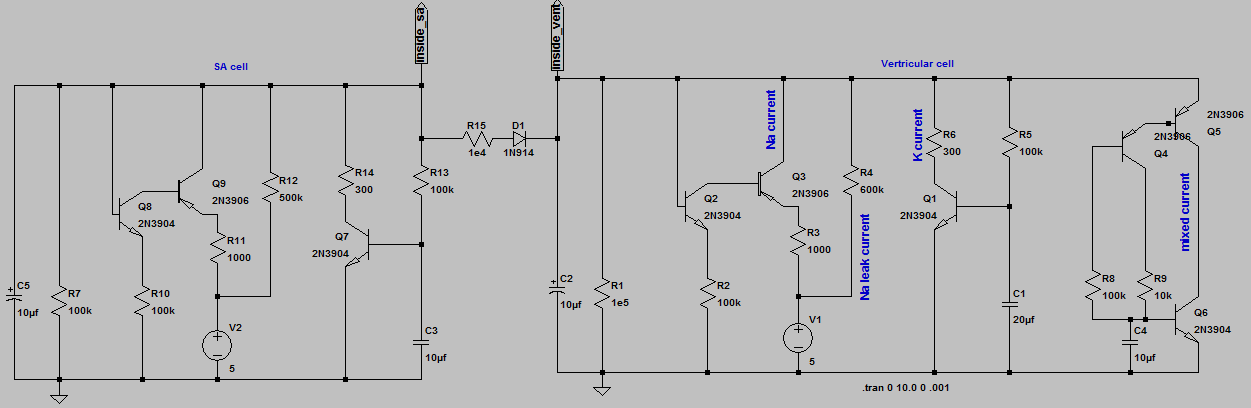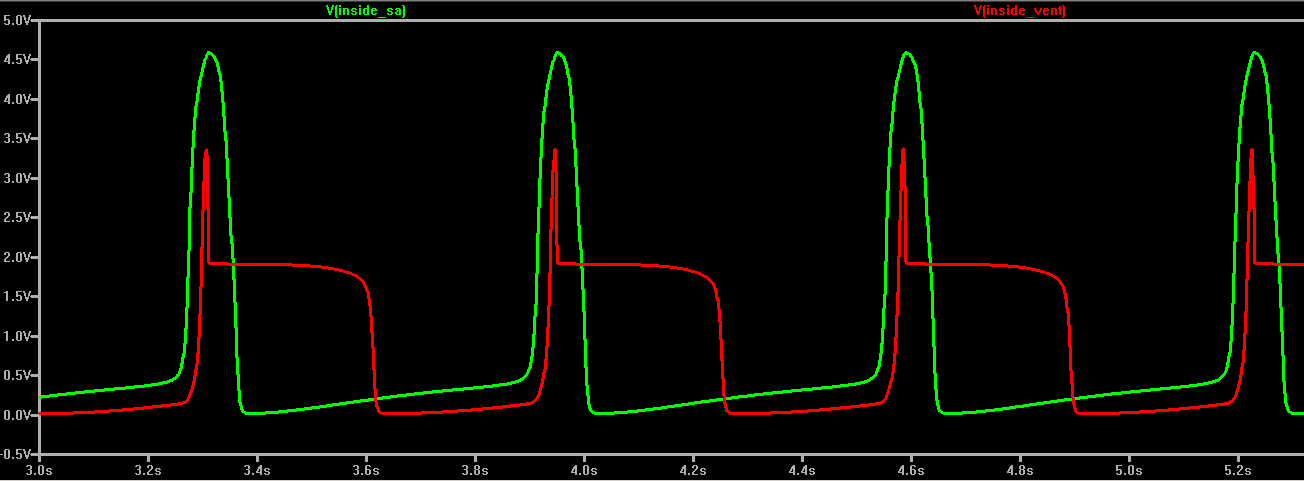## SA and Ventricular cell models.

Introduction.

In this exercise you will build a simulation of a cardiac ventricle cell with a fast depolarization, plateau, and repolarizaiton.

Procedure:

Since channels respond to voltage by changing their resistance, we will be measuring the curves of current versus voltage for several circuits. If the circuit were just a resistance, then plotting current versus voltage would be linear with a slope of 1/R (since I=V/R). Transistors allow us to design circuits with very nonlinear I-V curves, just like the biological ion channels we want to model.

Assignment:
Note that in this and every lab assignment the verb build means to construct a circuit on the protoype board with actual parts.
The verb simulate means use LTspice to mathematically simulate the circuit.

1. Download and simulate SA and ventricular cell shown below. You can vary the rate of the SA cell by changing R12 and the ventricular cell (spontaneous rate) by changing R4. The ability of the SA cell to drive (and synchronize) the ventricle depends on SA rate, ventricular spontaneous rate, and coupling resistance R15. Explore the relationship between these variables in simulation. For instance: change R15 to 1e9 ohms. Do the cells synchronize? If the ventricular rate is higher than the SA rate, can you get irregular beats?2. Build a ventricular cell and test it to make sure that it spontaneously pulses. Vary R4 to show this changes the rate.
3. Connect the ventricular model to the SA node you built last time using real hardware (not simulation). Show that for some combinations of R12, R4, and R15 you can get 1:1 and 2:1 phase locking with the real circuit. Can you produce an irregular rhythm (this would be bad in real life!).

This exercise is part of the next several lab assignments. There will no written lab report from this exercise, but you will need the data from this lab when you do the writeup at the end of lab 4.

August 17, 2011 Copyright Cornell university